# E3B water model

The E3B (explicit three-body) model of water.

## Parameters

The E3B potential is given by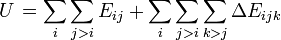$\left. U \right.= \sum_i \sum_{j>i} E_{ij} + \sum_i \sum_{j>i}\sum_{k>j} \Delta E_{ijk}$

where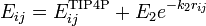$E_{ij} = E_{ij}^{\mathrm{TIP4P}} + E_2 e^{-k_2r_{ij}}$

where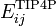$E_{ij}^{\mathrm{TIP4P}}$ represents the TIP4P model of water,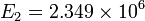$E_2 = 2.349\times10^6$ kJ/mol,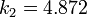$k_2 = 4.872$ Å-1, and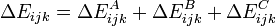$\Delta E_{ijk} =\Delta E_{ijk}^A + \Delta E_{ijk}^B + \Delta E_{ijk}^C$

where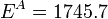$E^A = 1745.7$ kJ/mol,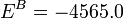$E^B = -4565.0$ kJ/mol, and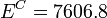$E^C = 7606.8$ kJ/mol.